UPSC  >  Letter Series- Introduction and Examples (with Solutions), Logical Reasoning

# Letter Series- Introduction and Examples (with Solutions), Logical Reasoning Notes | Study CSAT Preparation for UPSC CSE - UPSC

## Document Description: Letter Series- Introduction and Examples (with Solutions), Logical Reasoning for UPSC 2022 is part of CSAT Preparation for UPSC CSE preparation. The notes and questions for Letter Series- Introduction and Examples (with Solutions), Logical Reasoning have been prepared according to the UPSC exam syllabus. Information about Letter Series- Introduction and Examples (with Solutions), Logical Reasoning covers topics like Introduction, Examples and Letter Series- Introduction and Examples (with Solutions), Logical Reasoning Example, for UPSC 2022 Exam. Find important definitions, questions, notes, meanings, examples, exercises and tests below for Letter Series- Introduction and Examples (with Solutions), Logical Reasoning.

Introduction of Letter Series- Introduction and Examples (with Solutions), Logical Reasoning in English is available as part of our CSAT Preparation for UPSC CSE for UPSC & Letter Series- Introduction and Examples (with Solutions), Logical Reasoning in Hindi for CSAT Preparation for UPSC CSE course. Download more important topics related with notes, lectures and mock test series for UPSC Exam by signing up for free. UPSC: Letter Series- Introduction and Examples (with Solutions), Logical Reasoning Notes | Study CSAT Preparation for UPSC CSE - UPSC
 1 Crore+ students have signed up on EduRev. Have you?

Introduction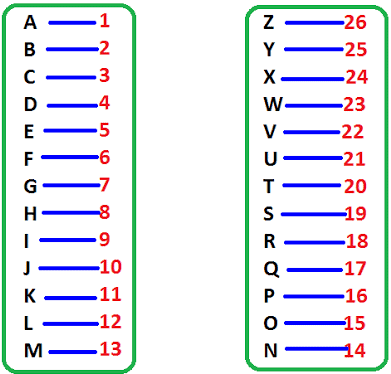• From the above diagram, you can easily see the position and opposite word of each alphabet.
Example: Position of D is 4 and opposite letter to D is W and position of W is 23.
• Position of J is 10 and opposite letter of J is Q and position of Q is 17 and so on

Examples

Find the letter in place of the question mark (?) in the series given below:

Q1. B, F, ?, N, R

a) G

b) K

c) J

d) L

e) None of The Above

Ans: Option C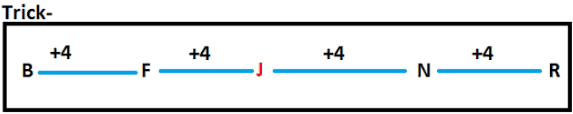Q2. P, ?, J, G, D, A

a) Q

b) N

c) K

d) M

e) None of The Above

Ans: Option D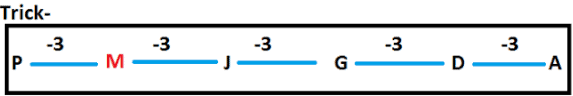Q3. ?, E, G, J, N

a) A

b) B

c) D

d) Z

e) None of The Above

Ans: Option C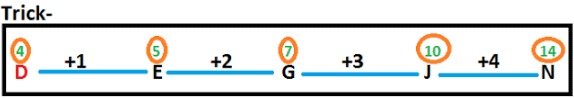Q4. X, F, Y, G, ?, H

a) Z

b) A

c) B

d) Y

e) None of The Above

Ans: Option A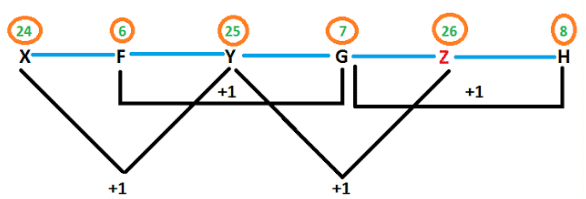Q5. B, B, A, D, ?, F

a) B

b) A

c) Z

d) C

e) None of The Above

Ans: Option C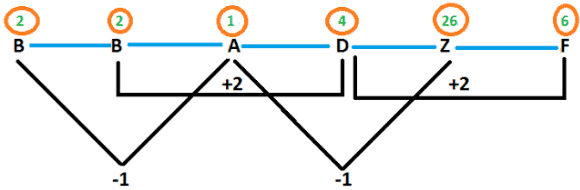Question for Letter Series- Introduction and Examples (with Solutions), Logical Reasoning
Try yourself:ZA5, Y4B, XC6, W3D, _____

Q.6. In this series, you will be looking at both the letter pattern and the number pattern. Fill the blank in the middle of the series or end of the series.

1. SCD, TEF, UGH, ____, WKL

A.   CMN

B.   UJI

C.   VIJ

D.   IJT

Explanation: There are two alphabetical series here. The first series is with the first letters only: STUVW. The second series involves the remaining letters: CD, EF, GH, IJ, KL.

Q.7. B2CD, _____, BCD4, B5CD, BC6D

A.    B2C2D

B.    BC3D

C.    B2C3D

D.    BCD7

Explanation: Because the letters are the same, concentrate on the number series, which is a simple 2, 3, 4, 5, 6 series, and follows each letter in order.

Question for Letter Series- Introduction and Examples (with Solutions), Logical Reasoning
Try yourself:QPO, NML, KJI, _____, EDC

Q.8. FAG, GAF, HAI, IAH, ____

A.    JAK

B.    HAL

C.    HAK

D.    JAI

Explanation: The middle letters are static, so concentrate on the first and third letters. The series involves an alphabetical order with a reversal of the letters. The first letters are in alphabetical order: F, G, H, I , J. The second and fourth segments are reversals of the first and third segments. The missing segment begins with a new letter.

Q.9. ELFA, GLHA, ILJA, _____, MLNA

A.    OLPA

B.    KLMA

C.    LLMA

D.    KLLA

Explanation: The second and fourth letters in the series, L and A, are static. The first and third letters consist of an alphabetical order beginning with the letter E.

Q.10. CMM, EOO, GQQ, _____, KUU

A.    GRR

B.    GSS

C.    ISS

D.    ITT

Explanation: The first letters are in alphabetical order with a letter skipped in between each segment: C, E, G, I, K. The second and third letters are repeated; they are also in order with a skipped letter: M, O, Q, S, U.

Question for Letter Series- Introduction and Examples (with Solutions), Logical Reasoning
Try yourself:JAK, KBL, LCM, MDN, _____

Q.11. BCB, DED, FGF, HIH, ___

A.    JKJ

B.    HJH

C.    IJI

D.    JHJ

Explanation: This series consists of a simple alphabetical order with the first two letters of all segments: B, C, D, E, F, G, H, I, J, K. The third letter of each segment is a repetition of the first letter.

Q.12. QAR, RAS, SAT, TAU, _____

A.    UAV

B.    UAT

C.    TAS

D.    TAT

Explanation: In this series, the third letter is repeated as the first letter of the next segment. The middle letter, A, remains static. The third letters are in alphabetical order, beginning with R.

The document Letter Series- Introduction and Examples (with Solutions), Logical Reasoning Notes | Study CSAT Preparation for UPSC CSE - UPSC is a part of the UPSC Course CSAT Preparation for UPSC CSE.
All you need of UPSC at this link: UPSC

## CSAT Preparation for UPSC CSE

72 videos|64 docs|92 tests
 Use Code STAYHOME200 and get INR 200 additional OFF

## CSAT Preparation for UPSC CSE

72 videos|64 docs|92 tests

### How to Prepare for UPSC

Read our guide to prepare for UPSC which is created by Toppers & the best Teachers

Track your progress, build streaks, highlight & save important lessons and more!

,

,

,

,

,

,

,

,

,

,

,

,

,

,

,

,

,

,

,

,

,

,

,

,

;